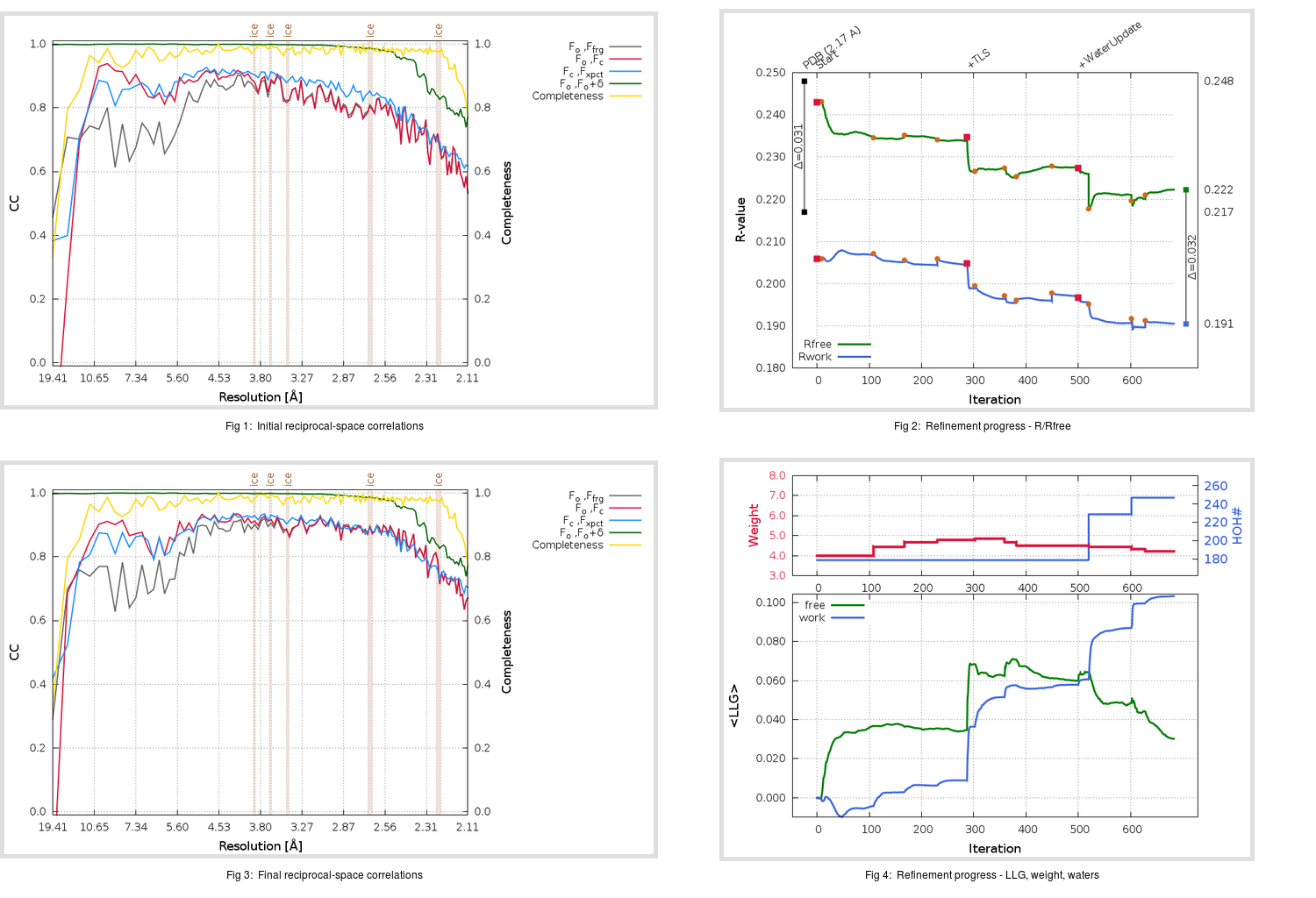Content:

```    Diffraction limits & principal axes of ellipsoid fitted to diffraction cut-off surface:
2.065         0.8627   0.0000   0.5057       0.989 a* + 0.151 c*
2.087         0.0000   1.0000   0.0000       b*
2.024        -0.5057   0.0000   0.8627      -0.727 a* + 0.687 c*
```

## Deposited

` `
 Date deposited Date data collection Resolution R, Rfree 20060511 20041017 2.17 0.2090 0.2480

Molprobity (CCP4 7.0 version) summary:

```Ramachandran outliers =   0.33 %
favored =  98.03 %
Rotamer outliers      =   6.08 %
C-beta deviations     =     3
Clashscore            =   7.03
RMS(bonds)            =   0.0111
RMS(angles)           =   1.31
MolProbity score      =   1.98
Resolution            =   2.17
R-work                =   0.2090
R-free                =   0.2480
```

```Number of waters      =   179

<B> (all atoms) =   35.62 ( sd =    8.00 ) for       2549 non-hydrogen atoms
<B>   (protein) =   35.18 ( sd =    7.69 ) for       2370 non-hydrogen atoms
<B>     (water) =   41.50 ( sd =    9.57 ) for        179 non-hydrogen atoms
<B>    (others) =    0.00 ( sd =    0.00 ) for          0 non-hydrogen atoms

B min/max       (all non-hydrogen atoms) =   15.29 /   76.23
B min/max   (protein non-hydrogen atoms) =   15.29 /   61.93
B min/max     (water non-hydrogen atoms) =   20.18 /   76.23
B min/max     (other non-hydrogen atoms) =    0.00 /    0.00
```

## BUSTER (re-)refinement

` `

Molprobity (CCP4 7.0 version) summary:

```Ramachandran outliers =   0.33 %
favored =  97.37 %
Rotamer outliers      =   4.94 %
C-beta deviations     =     0
Clashscore            =   2.77
RMS(bonds)            =   0.0113
RMS(angles)           =   1.57
MolProbity score      =   1.72
Resolution            =   2.10
R-work                =   0.1906
R-free                =   0.2224
```

```Number of waters      =   247

<B> (all atoms) =   41.03 ( sd =    9.96 ) for       2617 non-hydrogen atoms
<B>   (protein) =   40.14 ( sd =    9.46 ) for       2370 non-hydrogen atoms
<B>     (water) =   49.63 ( sd =   10.51 ) for        247 non-hydrogen atoms
<B>    (others) =    0.00 ( sd =    0.00 ) for          0 non-hydrogen atoms

B min/max       (all non-hydrogen atoms) =   23.33 /   91.75
B min/max   (protein non-hydrogen atoms) =   23.33 /   83.58
B min/max     (water non-hydrogen atoms) =   23.94 /   91.75
B min/max     (other non-hydrogen atoms) =    0.00 /    0.00
```

Refinement progression:Results:

` `
 File Remark 2GZ9_aB_refine.01_03_refine.pdb.gz exact refinement commands are in header 2GZ9_aB_refine.01_03_refine.mtz.gz including original deposited data and several re-refinement map coefficients 2GZ9_aB_refine.01_03_BUSTER_model.cif.gz including any non-standard compound restraints 2GZ9_aB_refine.01_03_BUSTER_refln.cif.gz